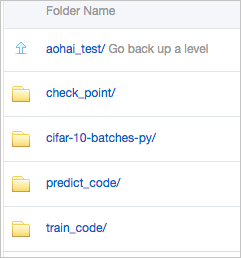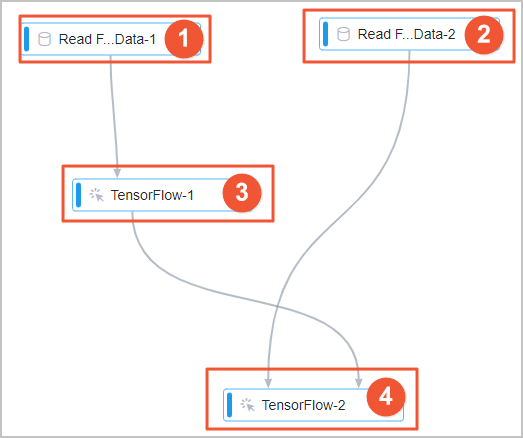This topic describes how to use TensorFlow to develop an image classification model in the Machine Learning Platform for AI console.

## Prerequisites

• An Object Storage Service (OSS) bucket is created. Machine Learning Platform for AI is authorized to access the OSS bucket. For more information, see Create buckets and Grant PAI the permissions to access OSS.
Notice When you create an OSS bucket, make sure that versioning is disabled for the bucket. Otherwise, model training may fail.
• GPU resources are enabled.
Note GPU resources that are based on MaxCompute are not supported.

## Background information

Due to the development of the Internet, a large amount of data related to images and voice is generated. Unstructured data poses a great challenge to data engineers due to the following reasons:
• Technical expertise is required to use deep learning algorithms.
• Computing resources such as GPU compute engines are pricey.

Machine Learning Studio provides a built-in image classification template that uses a deep learning framework. You can create an experiment from the template and use the experiment in scenarios such as content moderation, facial recognition, and object detection.

## Datasets

In the following sample experiment, the CIFAR-10 dataset is used. The dataset contains 60,000 color images of 32 × 32 pixels. The images are classified into 10 categories, including airplane, automobile, bird, cat, deer, dog, frog, horse, ship, and truck, as shown in the following figure. For more information about the download URLs of the dataset and relevant code, see CIFAR-10 dataset.In the experiment, the dataset is divided into a training dataset of 50,000 images and a prediction dataset of 10,000 images. The training dataset is further divided into five training batches. The entire prediction dataset is used as a test batch. Each batch is a separate file, as shown in the following figure.## Data preparation

Upload the dataset files and relevant code that are used in this experiment to an OSS bucket. For example, you can create a folder named aohai_test in an OSS bucket and four subfolders in the aohai_test folder, as shown in the following figure.The four subfolders are used for the following purposes:
• check_point: stores the model that is generated by the experiment.
Note After you create an experiment from the template in Machine Learning Studio, you must set the Checkpoint Output Directory/Model Input Directory parameter of the TensorFlow component to the path of an existing OSS folder. Otherwise, you cannot run the experiment. In this experiment, set the Checkpoint Output Directory/Model Input Directory parameter of the TensorFlow component to the path of the check_point subfolder.
• cifar-10-batches-py: stores the cifar-10-batcher-py file of the training dataset and the bird_mount_bluebird.jpg file of the prediction dataset.
• train_code: stores the cifar_pai.py file, which contains the training code.
• predict_code: stores the cifar_predict_pai.py file, which contains the prediction code.

## Use TensorFlow to develop an image classification model

1. Go to a Machine Learning Studio project.
1. Log on to the PAI console.
2. In the left-side navigation pane, choose Model Training > Visualized Modeling (Machine Learning Studio).
3. In the upper-left corner of the page, select the region where you want to use PAI.
4. Optional:In the search box on the Visualized Modeling page, enter the name of a project to search for the project.
5. Find the project and click Machine Learning in the Actions column.
2. Create an experiment.
1. In the left-side navigation pane, click Home.
2. On the Templates page, click Create below Tensorflow image classification.
3. In the New Experiment dialog box, set the following parameters. You can use the default settings.
Parameter Description
Name Enter TensorFlow image classification.
Project The name of the project to which the experiment belongs. You cannot change the value of this parameter.
Description Enter Use PAI-TensorFlow to build an image classification model.
Save To Select My Experiments.
4. Click OK.
5. Optional:Wait about 10 seconds and click Experiments in the left-side navigation pane.
6. Optional:Click TensorFlow image classification_XX below My Experiments.
My Experiments is the directory that stores the experiment and TensorFlow image classification_XX is the name of the experiment. In the experiment name, _XX is the ID that the system automatically creates for the experiment.
7. View the components of the experiment on the canvas, as shown in the following figure. The system automatically creates the experiment based on the template.Area Description
The component in this area reads the training data. The system automatically sets the OSS Data Path parameter of this component to the path of the training dataset that is used by the experiment. If you want to use another dataset, click the Read File Data-1 component on the canvas. In the Fields Setting panel on the right side, set the OSS Data Path parameter to the OSS path of the dataset that you want to use.
The component in this area reads the prediction data. The system automatically sets the OSS Data Path parameter of this component to the path of the prediction dataset that is used by the experiment. If you want to use another dataset, click the Read File Data-2 component on the canvas. In the Fields Setting panel on the right side, set the OSS Data Path parameter to the OSS path of the dataset that you want to use.
The component in this area trains the model by using TensorFlow. Set the Checkpoint Output Directory/Model Input Directory parameter of this component and use the default settings for other parameters. The following parameters specify the OSS paths of the corresponding files or folders:
• Python Code Files: the OSS path of the cifar_pai.py file.
• Data Source Directory: the OSS path of the cifar-10-batches-py folder. The system automatically synchronizes data from the parent node named Read File Data-1.
• Checkpoint Output Directory/Model Input Directory: the OSS path of the check_point folder, which stores the generated model.
The component in this area generates the prediction result. Set the Checkpoint Output Directory/Model Input Directory parameter of this component and use the default settings for other parameters. The following parameters specify the OSS paths of the corresponding files or folders:
• Python Code Files: the OSS path of the cifar_predict_pai.py file.
• Data Source Directory: the OSS path of the cifar-10-batches-py folder. The system automatically synchronizes data from the parent node named Read File Data-2.
• Checkpoint Output Directory/Model Input Directory: the OSS path of the check_point folder, which stores the generated model. You must set this parameter to the same value as the Checkpoint Output Directory/Model Input Directory parameter of the component that trains the model by using TensorFlow.
3. Run the experiment and view the result.
1. In the upper-left corner of the canvas, click Run.
2. After the experiment stops running, you can view the prediction result in the OSS path that is specified by Checkpoint Output Directory/Model Input Directory.

## Training code

This section describes the key code in the cifar_pai.py file.
• The following code can be used to train a convolutional neural network (CNN) model for image classification:
``````network = input_data(shape=[None, 32, 32, 3],
data_preprocessing=img_prep,
data_augmentation=img_aug)
network = conv_2d(network, 32, 3, activation='relu')
network = max_pool_2d(network, 2)
network = conv_2d(network, 64, 3, activation='relu')
network = conv_2d(network, 64, 3, activation='relu')
network = max_pool_2d(network, 2)
network = fully_connected(network, 512, activation='relu')
network = dropout(network, 0.5)
network = fully_connected(network, 10, activation='softmax')
loss='categorical_crossentropy',
learning_rate=0.001)``````
• The following code can be used to generate a model named model.tfl:
``````    model = tflearn.DNN(network, tensorboard_verbose=0)
model.fit(X, Y, n_epoch=100, shuffle=True, validation_set=(X_test, Y_test),
show_metric=True, batch_size=96, run_id='cifar10_cnn')
model_path = os.path.join(FLAGS.checkpointDir, "model.tfl")
print(model_path)
model.save(model_path)``````

## Prediction code

This section describes the key code in the cifar_predict_pai.py file. The system reads the bird_bullocks_oriole.jpg image file and resizes the image to 32 × 32 pixels. Then, the system passes the image to the model.predict function. The output of the function is the weights of the 10 categories based on which the image is recognized: ['airplane','automobile','bird','cat','deer','dog','frog','horse','ship','truck']. The system returns the category that has the highest weight as the prediction result.
``````    predict_pic = os.path.join(FLAGS.buckets, "bird_bullocks_oriole.jpg")